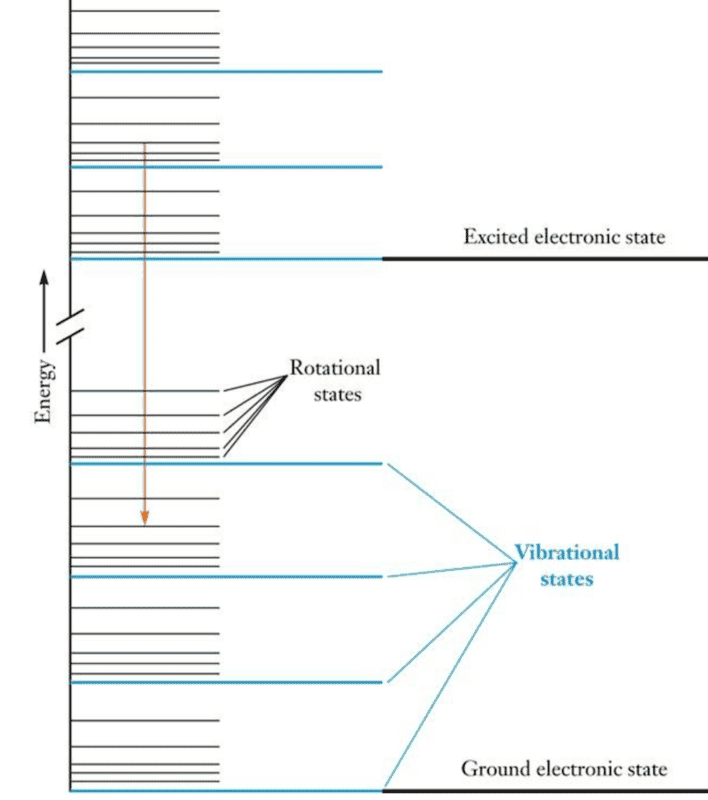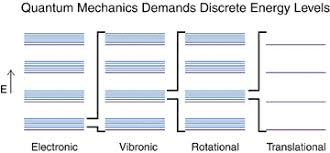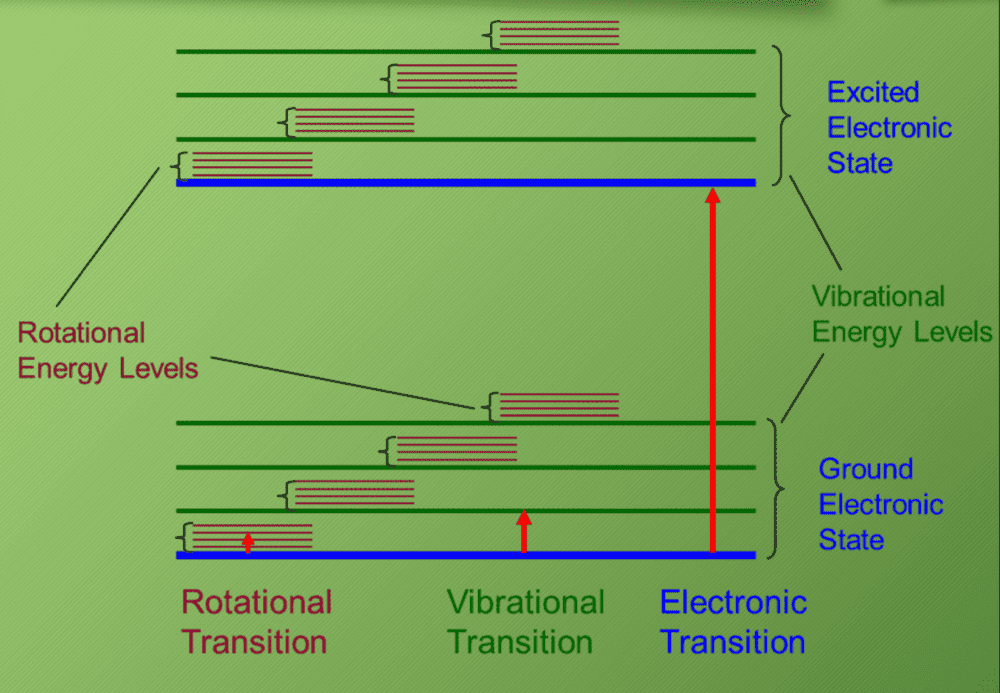# Interpreting Energy diagrams for polyatomic molecules

fog37
TL;DR Summary
understand energy levels in energy diagram for compounds
Hello,
I am trying to correctly interpret the energy diagram below.

For example, a diatomic molecule can translate (kinetic energy), rotate (rotational energy), vibrate (vibrational energy). Each different type of energy is quantized and has associated modes (also called states). The rotational, vibrational, translational energy states/levels/modes pertain to the molecule as a whole. On the other hand, electronic energy levels are electrostatic potential energy levels (not kinetic energy) and pertain to the electrons and nuclei.

The vertical axis in the diagram, labelled Energy, therefore indicates energy in general, correct?Based on the next figure below, what does it mean that an electronic energy level is comprised by several vibrational energy levels which are, in turn, comprised by many rotational energy levels? I know that when multiple molecules connect together, energy bands are formed instead of discrete and sharp energy levels. A band is formed by many discrete energy levels slightly separated from each other.

Do rotational/vibrational states get excited when a molecules absorbs external energy that does not match the difference between electronic energy levels?Last edited:

Homework Helper
Gold Member
At each electronic energy level, the total energy is still a sum of the electronic, rotational, vibrational and translational. Those fine energy levels superimposed on the electronic states reflect those states as well. You may need to adjust your understanding of “where” the energy resides. Electronic energy levels are for the bond within the whole molecule and the vibrational, rotational and translational energies go along for that ride as well within each bond.

Do rotational/vibrational states get excited when a molecules absorbs external energy that does not match the difference between electronic energy levels?
. Those types of energy that molecules absorb relate to that fine structure you see described. Vibrational energy is coupled into the vibrational bands (infrared energy), translational energy into the translational bands (temperature) and rotational energy by the rotational bands ( microwave energy).

Last edited:
•fog37
fog37
Thank you chemisttree.

What do you mean that "Vibrational energy is coupled into the vibrational bands (infrared energy)..."

Coupled in what sense? I know that if the diatomic molecules absorbs energy equal to the the difference from ground state to first excited electronic energy state (thick blue lines), the molecule will move to that excited electronics state...Will the molecule be automatically excited into vibrational (red/green lines) and rotational states during that transition? I don't believe so.

From the figure below, if the absorbed energy is not equal to jump from blue line to blue line (longer red transition), then the molecule may be excited into vibrational or rotational states...How could it get excited into both types of states if it needs to land on a specific horizontal energy line?#### Attachments

Homework Helper
How could it get excited? By absorbing a photon of the right energy. It is perfectly possible to change rotational, vibrational and electronic states at the same time (though not without limit - there are selection rules). For example, in the diagram in your initial post, there is a red arrow (going downwards, so the molecule will emit a photon and lose energy) from a level in the upper electronic state with vibrational and rotational quantum numbers v' = 1 and J' = 3 to a level in the lower electronic state with v'' = 2 and J'' = 4. (Δv = ± 1 and ΔJ = ±1 are allowed.) Indeed, sometimes an electronic transition (between the ground vibrational states of two electronic states) which is forbidden by symmetry can be allowed if a vibrational mode of appropriate symmetry is simultaneously excited (i.e. between v'' = 0 and v' = 1); then we say that the transition is "vibronically allowed".

•chemisttree and BvU
Homework Helper
Wow, that was a quick "like"!

Had some 1972 lecture notes at hand ...